# (a) How much current flows in a 100 watt bulb when lit? Assume 120 volts and...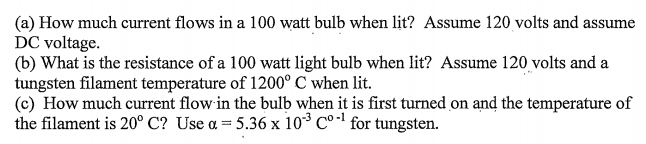(a) How much current flows in a 100 watt bulb when lit? Assume 120 volts and assume DC voltage. (b) What is the resistance of a 100 watt light bulb when lit? Assume 120 volts and a tungsten filament temperature of 1200° C when lit. (c) How much current flow in the bulb when it is first turned on and the temperature of the filament is 20° C? Use α-5.36 x 10-3 Co.1 for tungsten.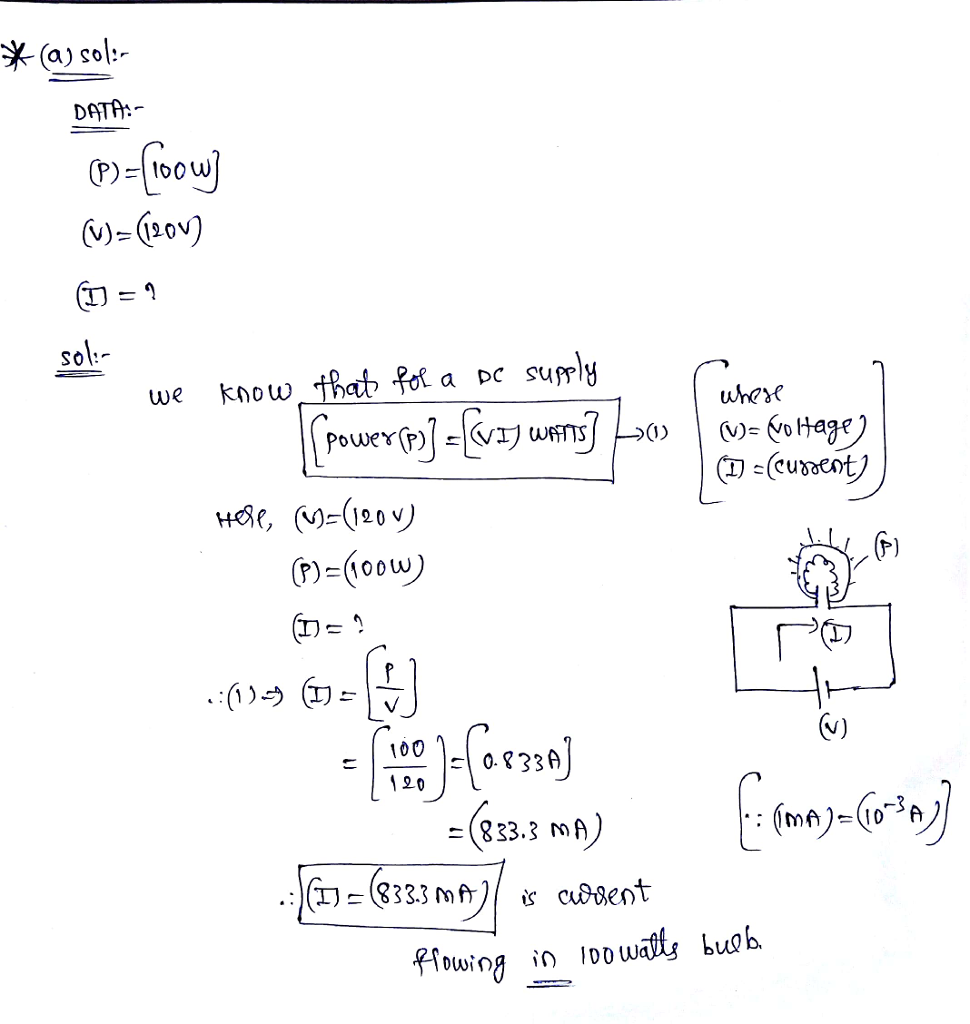#### Earn Coin

Coins can be redeemed for fabulous gifts.

Similar Homework Help Questions
• ### use kirchoffs rule if applicable A common flashlight bulb is rated at 0.300 A and 2.90...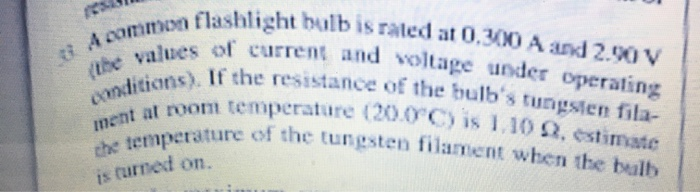use kirchoffs rule if applicable A common flashlight bulb is rated at 0.300 A and 2.90 V (the values of current and voltage under operating conditions). if the resistance of the bulbs tungsten filament at room temperature (20.0 degrees C) is 1.10( ), estimate the temperature of the tungsten Filament when the bulb is turned on. A common flashlight bulb is rated at 0.90 A and 2.90 V (the values of current and voltage under operating conditions). If the resistance...

• ### Review Con: Part A An incandescent light bulb uses a coiled filament of tungsten that is...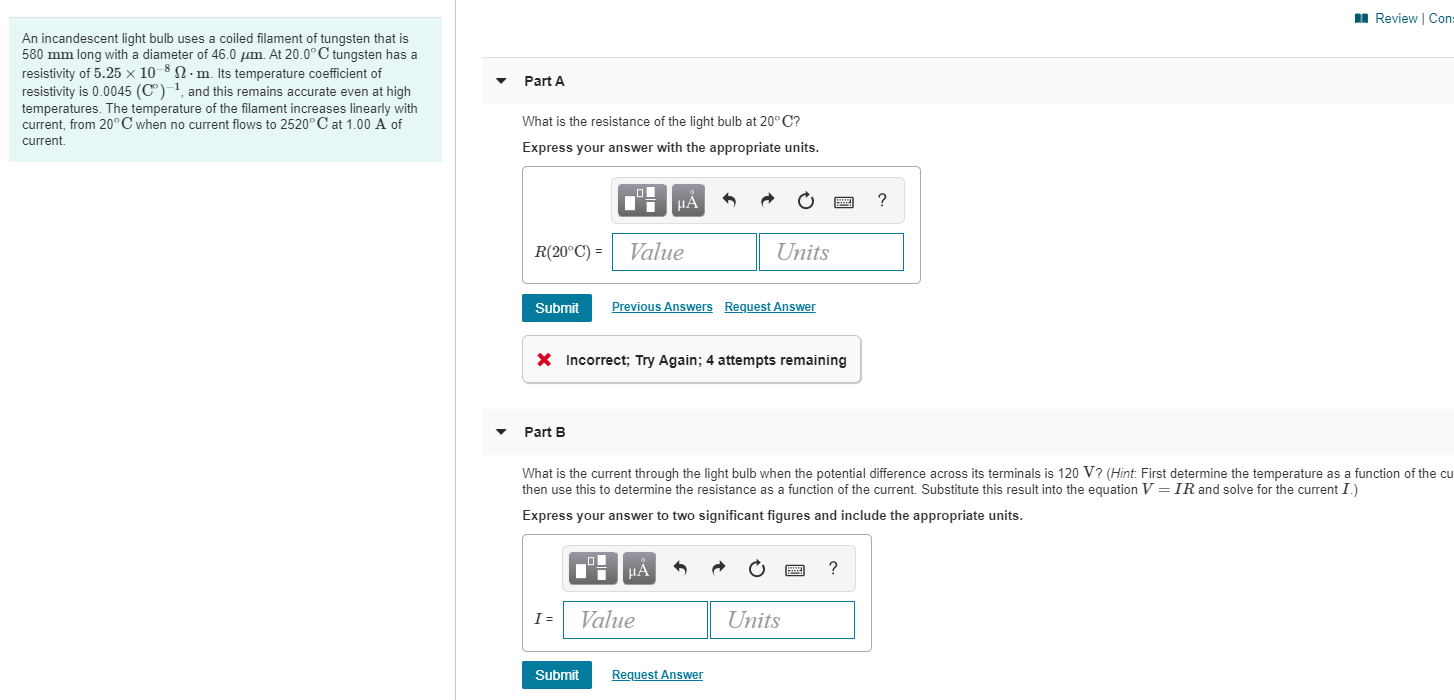Review Con: Part A An incandescent light bulb uses a coiled filament of tungsten that is 580 mm long with a diameter of 46.0 um. At 20.0°C tungsten has a resistivity of 5.25 x 1012.m. Its temperature coefficient of resistivity is 0.0045 (Cº) , and this remains accurate even at high temperatures. The temperature of the filament increases linearly with current, from 20°C when no current flows to 2520°C at 1.00 A of current What is the resistance of the...

• ### The filament of a 100 W (120 V) light bulb is a tungsten wire 0.035 mm...

The filament of a 100 W (120 V) light bulb is a tungsten wire 0.035 mm in diameter. At the filament's operating temperature, the resistivity is 5.0×10−7Ω⋅m. How long is the filament? L=? m

• ### ​ The filament of a 100 W (120 V) light bulb is a tungsten wire 0.035 mm in diameter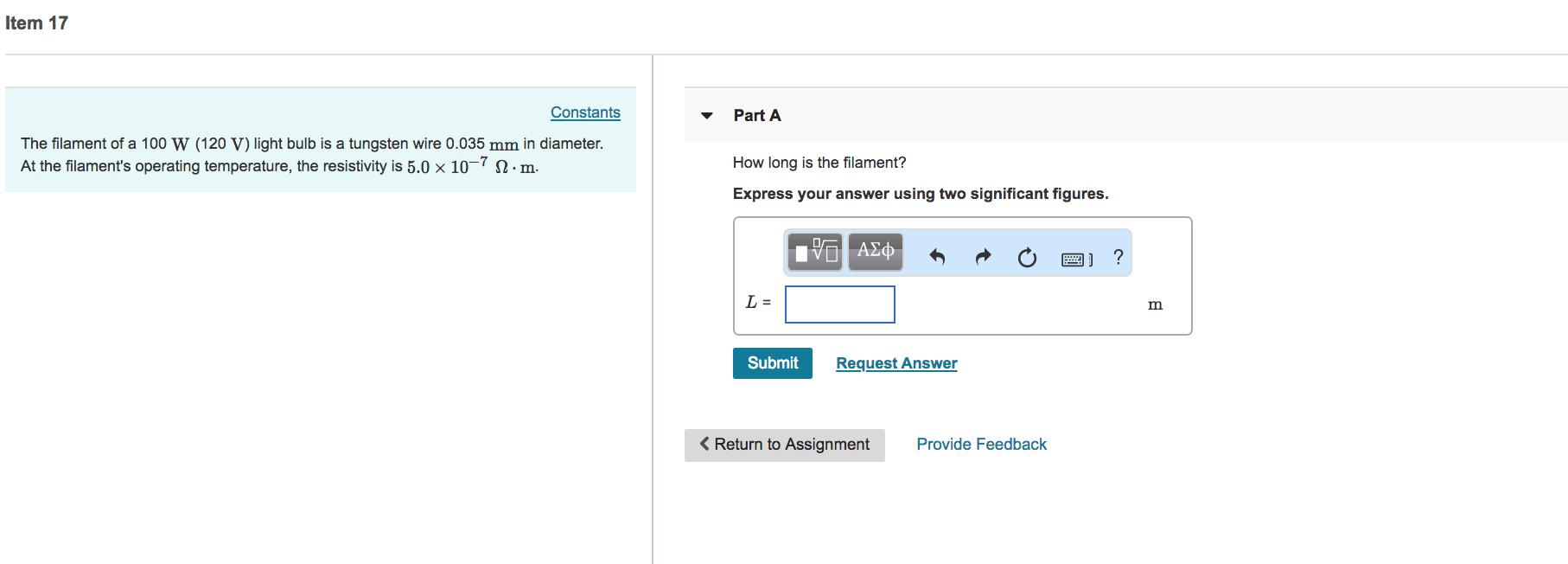The filament of a 100 W (120 V) light bulb is a tungsten wire 0.035 mm in diameter. At the filament's operating temperature, the resistivity is 5.0 × 10-7 Ω·m Part AHow long is the filament? Express your answer using two significant figures

• ### 1. The filament of a standard 100-W light bulb has a resistance of about 100 ohms....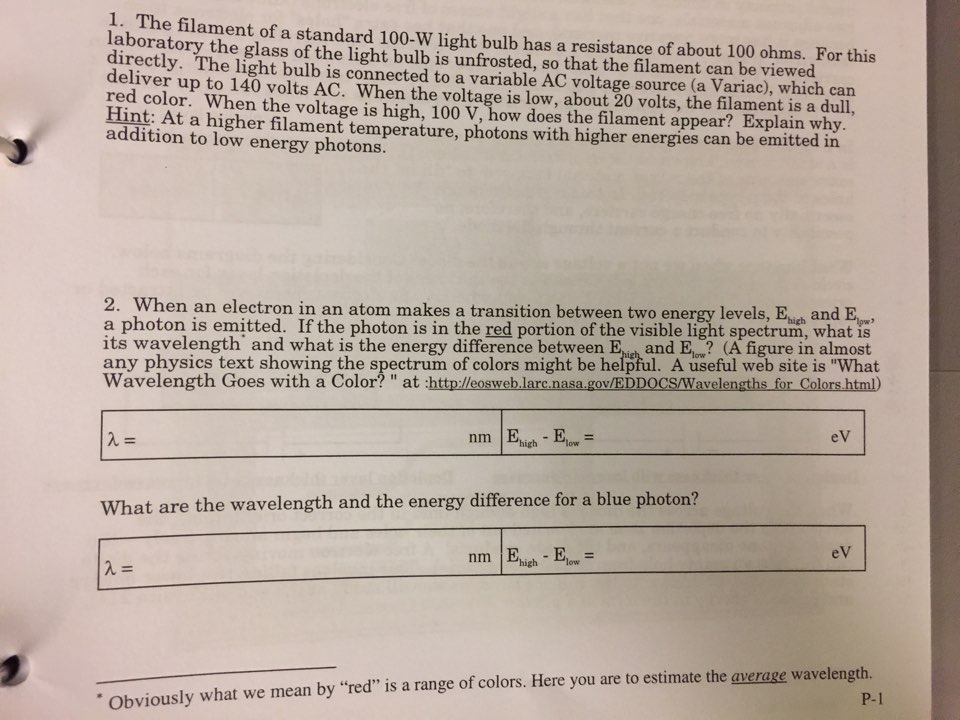1. The filament of a standard 100-W light bulb has a resistance of about 100 ohms. For this laboratory the glass of the light bulb is unfrosted, so that the filament can be viewed directly. The light bulb is connected to a variable AC voltage source (a Variac), which can deliver up to 140 volts AC. When the voltage is low, about 20 volts, the filament is a dull, red color. When the voltage is high, 100 V, how does...

• ### 4) a) What is the current in a 75 W the filament of a light bulb...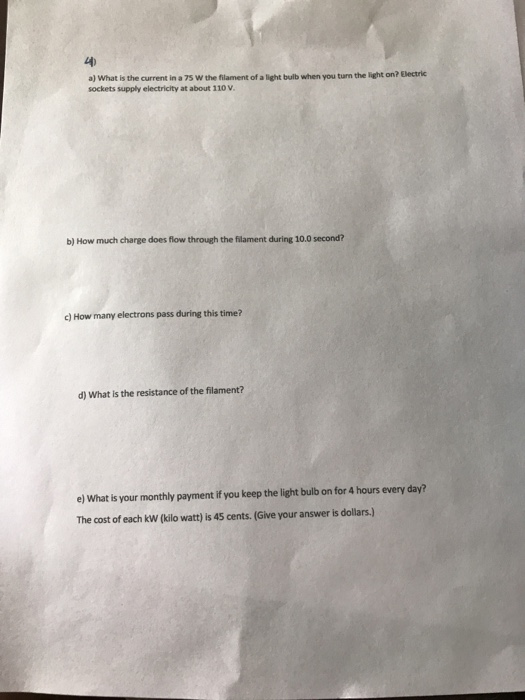4) a) What is the current in a 75 W the filament of a light bulb when you tum the light on? Electric sockets supply electricity at about 110 V b) How much charge does flow through the filament during 10.0 second? c) How many electrons pass during this time? d) What is the resistance of the filament? e) What is your monthly payment if you keep the light bulb on for 4 hours every day? The cost of each...

• ### Problem 8.3. The temperature of the tungsten filament in a 100 W light bulb is at 3000 C. Assume ...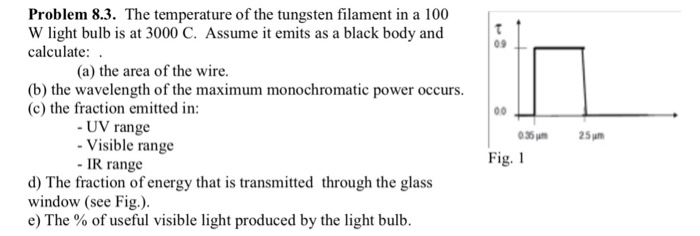Problem 8.3. The temperature of the tungsten filament in a 100 W light bulb is at 3000 C. Assume it emits as a black body and calculate: 09 (a) the area of the wire. wavelength of the max (c) the fraction emitted in: UV range Visible range 35 m25m Fig. 1 - IR range d) The fraction of energy that is transmitted through the glass window (see Fig.). e) The % of useful visible light produced by the light bulb....

• ### The power rating of a light bulb (such as a 100-W bulb) is the power it...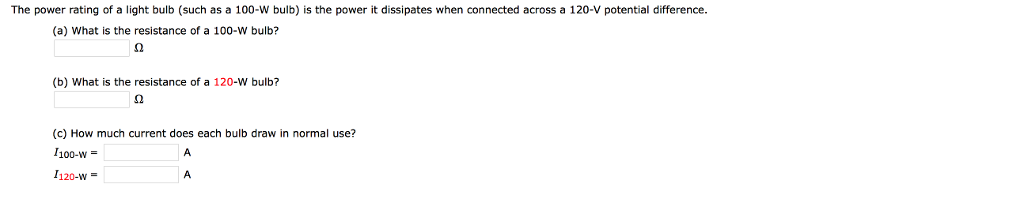The power rating of a light bulb (such as a 100-W bulb) is the power it dissipates when connected across a 120-V potential difference (a) What is the resistance of a 100-W bulb? (b) What is the resistance of a 120-W bulb? (c) How much current does each bulb draw in normal use? 1100-w = 1120-w-

• ### Light bulb A is marked "25 W 120 V" and light bulb B "100 W 120...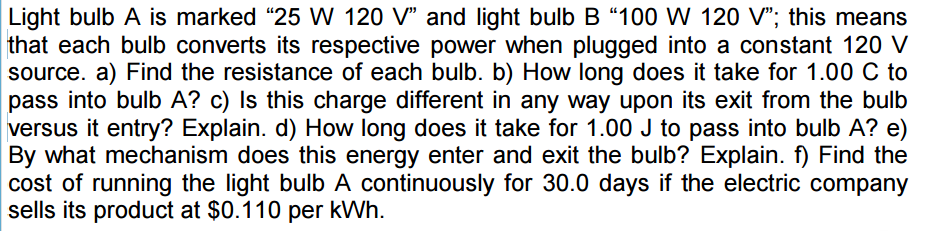Light bulb A is marked "25 W 120 V" and light bulb B "100 W 120 V"; this means that each bulb converts its respective power when plugged into a constant 120 V source, Find the resistance of each bulb, How long does it take for 1.00 C to pass into bulb A? Is this charge different in any way upon its exit from the bulb versus it entry? Explain, How long does it take for 1.00 J to pass...

• ### A light bulb is rated at 30 W when operated at 120 V. How much charge...

A light bulb is rated at 30 W when operated at 120 V. How much charge enters (and leaves) the light bulb in 1.0 min? a. 17 C b. 15 C c. 14 C d. 13 C e. 60 C